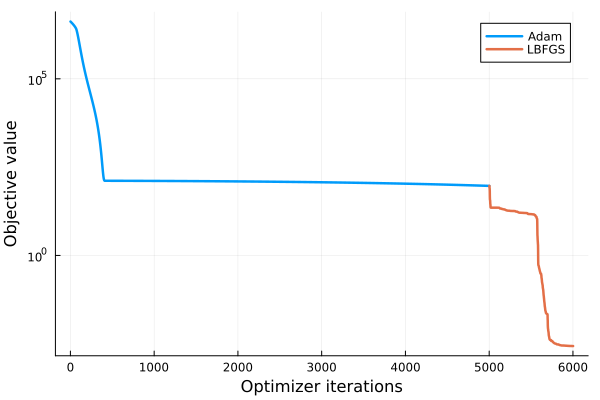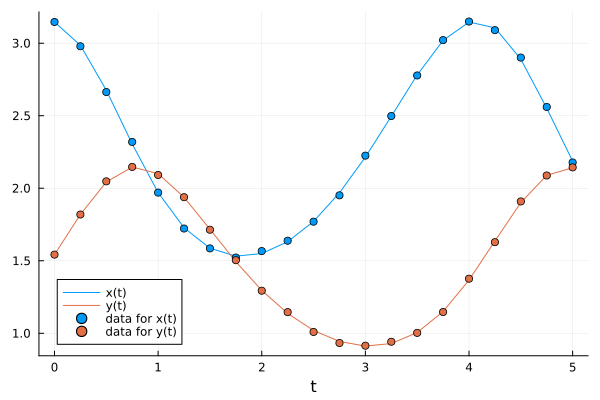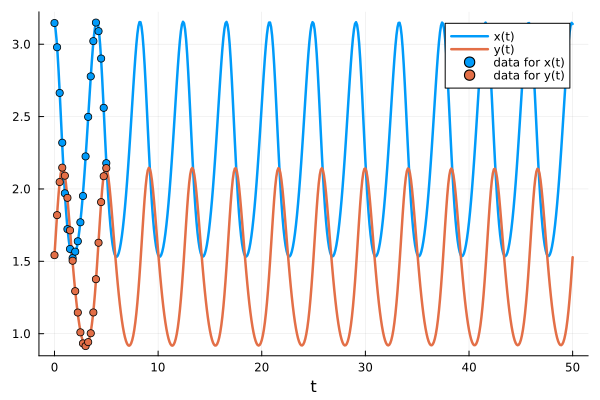# Model Autocomplete

In this tutorial, we show how to use PumasQSP to extend a partial model via the auto-complete function to find the missing physics.

## Motivation

When calibrating models to data, we often not only encounter uncertainties about the parameter values but also about the structure of the model. This can happen no matter how hard we try to fit our model to the data and could point out that we need to adjust the equations of the model. Universal Differential Equations (UDEs) address issue. Starting with a partial model, they find the minimal mechanistic extension that would provide a better fit.

The model we consider in this tutorial is the Lotka-Volterra model. Its equations are given by:

\begin{aligned} \frac{dx}{dt} &= \alpha x - \beta x y \\ \frac{dy}{dt} &= -\delta y + \gamma x y \\ \end{aligned}

It is a model of rabbits and wolves. $\alpha x$ is the exponential growth of rabbits in isolation, $-\beta x y$ and $\gamma x y$ are the interaction effects of wolves eating rabbits, and $-\delta y$ is the term for how wolves die hungry in isolation.

Now assume that we have never seen rabbits and wolves in the same room. We only know the two effects $\alpha x$ and $-\delta y$. Can we use Scientific Machine Learning to automatically discover an extension to what we already know? That is what we will solve with the universal differential equation.

## Julia environment

For this tutorial we will need the following packages:

ModuleDescription
PumasQSPPumasQSP is used to formulate our problem and perform automated model discovery
ModelingToolkitThe symbolic modeling environment
OrdinaryDiffEq (DifferentialEquations.jl)The numerical differential equation solvers
DataSetsWe will load our experimental data from datasets on JuliaHub
CSVHandling delimited text data
DataFramesHandling tabular data manipulation
OptimizationOptimisersThe optimization solver package with Adam
OptimizationOptimJLThe optimization solver package with LBFGS
DataDrivenSparseThe sparse regression symbolic regression solvers
StableRNGsStable random seeding
PlotsThe plotting and visualization library

Besides that we'll also need the following Julia standard libraries:

ModuleDescription
LinearAlgebraRequired for the norm function
StatisticsRequired for the mean function

First we prepare the environment by listing the packages we are using within the example.

using PumasQSP
using ModelingToolkit
using OrdinaryDiffEq
using DataSets
using CSV
using DataFrames
using OptimizationOptimJL: LBFGS
using StableRNGs
using LinearAlgebra
using Statistics
using Plots
Plots.GRBackend()

## Model setup

First, we define the incomplete model using ModelingToolkit.

# Model states and their default values
@variables begin
t
x(t) = 5.0
y(t) = 5.0
end

# Model parameters and their default values
@parameters  begin
α = 1.3
δ = 1.8
end

# Differential operator
D = Differential(t)

# Differential equations
eqs = [
D(x) ~ α * x,
D(y) ~ - δ * y,
]

# Model definition
@named incomplete_model = ODESystem(eqs)
\begin{align} \frac{\mathrm{d} x\left( t \right)}{\mathrm{d}t} =& \alpha x\left( t \right) \\ \frac{\mathrm{d} y\left( t \right)}{\mathrm{d}t} =& - \delta y\left( t \right) \end{align}

Second, we read in the training data (that corresponds to the correct model data + some small normally distributed noise).

# Load the data
training_dataset = dataset("pumasqsptutorials/lotka_data")
data = open(IO, training_dataset) do io
end
21×3 DataFrame
Rowtimestampx(t)y(t)
Float64Float64Float64
10.03.146391.54233
20.252.978951.81893
30.52.663412.04754
40.752.319332.14718
51.01.969962.09154
61.251.722331.93848
71.51.585251.71391
81.751.522221.50399
92.01.566851.29362
102.251.638341.14605
112.51.769521.00918
122.751.951160.932905
133.02.224750.914375
143.252.497910.941644
153.52.777681.00284
163.753.021391.14662
174.03.149391.37638
184.253.089991.6282
194.52.900961.90895
204.752.560372.08787
215.02.177862.14388

Lastly, we bring model and data together in a Trial object and build the InverseProblem with the neural component via the neural_network keyword argument.

# Trial definition
trial = Trial(training_dataset, incomplete_model;
u0 = [x => data."x(t)", y => data."y(t)"],
alg = Vern7(),
abstol = 1e-6,
reltol = 1e-6
)
# Construct inverse problem
prob = InverseProblem(trial, incomplete_model, []; neural_network = multi_layer_feed_forward(2, 2), nn_rng = StableRNG(1111))
InverseProblem for incomplete_model with one trial optimizing 0 parameters.


The neural_network keyword argument accepts a Lux.jl model. For ease of use, PumasQSP provides functions that can build such models easily, such as multi_layer_feed_forward. As our model has two states, the input and output of the neural network will be two.

## Step 1: Training the neural network

The training of the neural network is done by calibrating the inverse problem in two stages, i.e. we forward the first calibration result using the Adam() optimizer to the latter calibration call using the LBFGS() optimizer.

res1 = calibrate(prob, SingleShooting(; maxiters = 5000, optimizer = Adam()))
res2 = calibrate(res1, SingleShooting(; maxiters = 1000, optimizer = LBFGS()))
Calibration result computed in 2 minutes and 43 seconds. Final objective value: 0.00271634.
Optimization ended with Failure Return Code.



We visualize the convergence of the two optimizers.

## pl_losses
pl_losses = convergenceplot([res1, res2], yscale = :log10, lw = 2.5)We also visualize the learnt model together against the training data to estimate the accuracy of the neural network.

## pl_trajectory
pl_trajectory = plot(trial, prob, res2, show_data = true, legend = :bottomleft)## Step 2: Learning a symbolic representation from the neural network

We can now use the training results to autocomplete our model with symbolic terms that match the data. This can be done with the autocomplete function, which is compatible with DataDrivenDiffEq algorithms. For this example we will use the ADMM algorithm from DataDrivenSparse.jl and we will assume that our missing terms come from a polynomial basis of degree 4. In order to improve the accuracy of the symbolic interpretation, we process the neural network predictions in batches and we normalize the results using the Z-score transformation.

λ = exp10.(-3:0.01:3)
data_processing = DataProcessing(split = 0.9, batchsize = 30, shuffle = true)
res = autocomplete(res2, alg;
basis_generator = (polynomial_basis, 4),
digits = 1,
normalize = DataNormalization(ZScoreTransform),
data_processing)
Calibration result computed in 21 seconds and 698 milliseconds. Final objective value: 0.0032404.
Optimization ended with Success Return Code and returned.

┌─────────────┬─────────────┬───────────┬───────────┬────────────┬──────────┐
│          p₁ │          p₂ │        p₃ │        p₄ │         p₅ │       p₆ │
├─────────────┼─────────────┼───────────┼───────────┼────────────┼──────────┤
│ 0.000761669 │ -0.00695412 │ -0.896389 │ 0.0065833 │ 0.00210341 │ 0.798034 │
└─────────────┴─────────────┴───────────┴───────────┴────────────┴──────────┘


The autocomplete function returns a calibration result as the new model parameters are calibrated to best match the data. We can extract the autocompleted model using get_model and retrieve the learnt equations.

learnt_model = get_model(res)
learnt_equations = equations(learnt_model)
\begin{align} \frac{\mathrm{d} x\left( t \right)}{\mathrm{d}t} =& p_1 + \alpha x\left( t \right) + p_2 y\left( t \right) + p_3 x\left( t \right) y\left( t \right) \\ \frac{\mathrm{d} y\left( t \right)}{\mathrm{d}t} =& p_4 + p_5 y\left( t \right) - \delta y\left( t \right) + p_6 x\left( t \right) y\left( t \right) \end{align}

We can also use this new model to make predictions outside of the training data by solving for a larger timespan.

t = only(get_trials(res))
plot(t, res, tspan = (0, 50.), saveat = 0.1, show_data = true, legend = :topright, lw = 2.5)